×#### Thank you for registering.

One of our academic counsellors will contact you within 1 working day.

Click to Chat

1800-1023-196

+91-120-4616500

CART 0

• 0

MY CART (5)

Use Coupon: CART20 and get 20% off on all online Study Material

ITEM
DETAILS
MRP
DISCOUNT
FINAL PRICE
Total Price: Rs.

There are no items in this cart.
Continue Shopping• Complete JEE Main/Advanced Course and Test Series
• OFFERED PRICE: Rs. 15,900
• View Details

```Chapter 14: Compound Interest Exercise – 14.4

Question: 1

The present population of a town is 28000. If it increases at the rate of 5% per annum, what will be its population after 2 years?

Solution: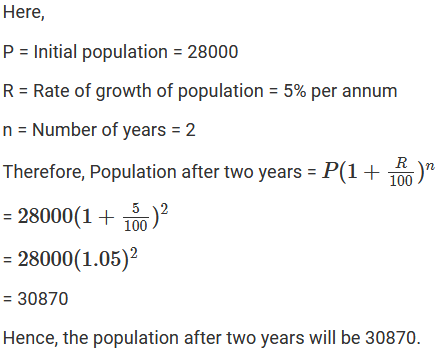Question: 2

The population of a city is 126000. If the annual birth rate and death rate are 5.5% and 9.5% respectively, calculate the population of city after 3 years.

Solution: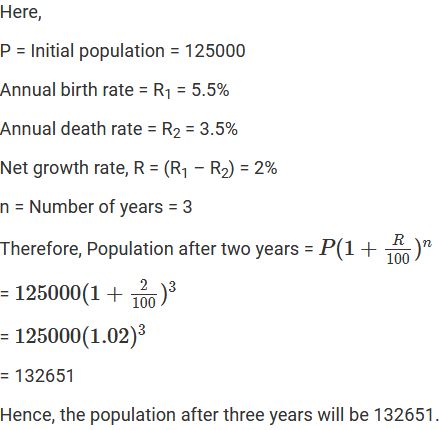Question: 3

The present population of a town is 25000. It grows at 4%, 5% and 8% during first year, second year and third year respectively. Find its population after 3 years.

Solution:Question: 4

Three years ago, the population of a town was 50000. If the annual increase during three successive years be at the rate of 4%, 5% and 3% respectively, find the present population.

Solution:Question: 5

There is a continuous growth in the population of a village at the rate of 5% per annum. If its present population is 9261, what it was 3 years ago?

Solution: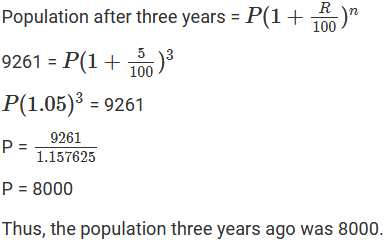Question: 6

In a factory the production of scooters rose to 46305 from 40000 in 3 years. Find the annual rate of growth of the production of scooters.

Solution: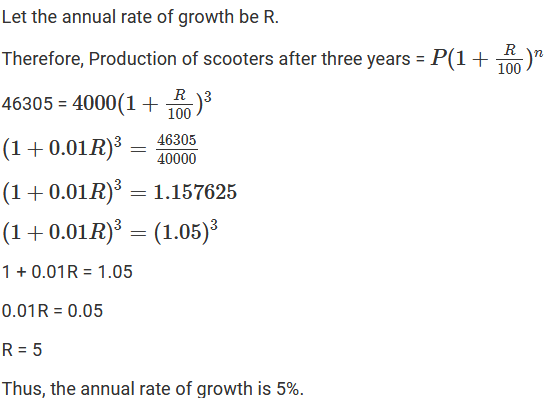Question: 7

The annual rate of growth in the population of a certain city is 8%. If its present population is 196830, what it was 3 years ago?

Solution:Question: 8

The population of a town increases at the rate of 50 per thousand. Its population after 2 years will be 22050. Find its present population.

Solution:Question: 9

The count of bacteria in a culture grows by 10% in the first hour, decreases by 8% in the second hour and again increases by 12% in the third hour. If the count of bacteria in the sample is 13125000, what will be the count of bacteria after 3 hours?

Solution: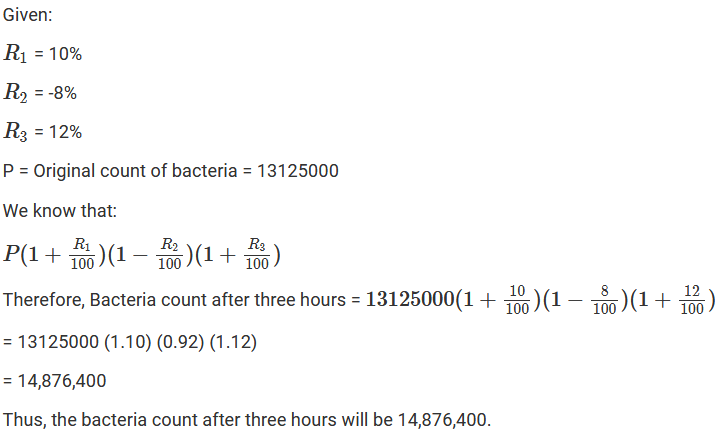Question: 10

The population of a certain city was 72000 on the last day of the year 1998. During next year it increased by 7% but due to an epidemic, it decreased by 10% in the following year. What was its population at the end of the year 2000?

Solution:Question: 11

6400 workers were employed to construct a river bridge in four years. At the end of the first-year, 25% workers were retrenched. At the end of the second year, 25% of those working at that time were retrenched. However, to complete the project in time, the number of workers was increased by 25% at the end of the third year. How many workers were working during the fourth year?

Solution:

Number of workers = 6,400

At the end of the first year, 25% of the workers were retrenched.

Therefore, 25% of 6,400 = 1,600

Number of workers at the end of the first year = 6,400 – 1600 = 4,800

At the end of the second year, 25% of those working were retrenched.

Therefore, 25% of 4,800 = 1,200

Number of workers at the end of the second year = 4,800 – 1200 = 3,600

At the end of the third year, 25% of those working increased.

Therefore, 25% of 3,600 = 900

Number of workers at the end of the third year = 3,600 + 900 = 4,500

Thus, the number of workers during the fourth year was 4,500.

Question: 12

Aman started a factory with an initial investment of its 100000. In the first year, he incurred a loss of 5%. However, during the second year, he earned a profit of 10% which is the third year rose to 12%. Calculate his net profit for the entire period of three years.

Solution: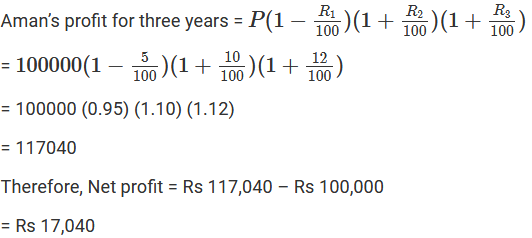Question: 13

The population of a town increases at the rate of 40 per thousand annually. If the present population be 175760, what was the population three years ago?

Solution: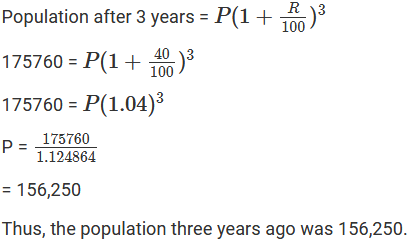Question: 14

The production of a mixed company in 1996 was 8000 mixies. Due to increase in demand, it increases its production by 15% in the next two years and after two years its demand decreases by 5%. What will be its production after 3 years?

Solution: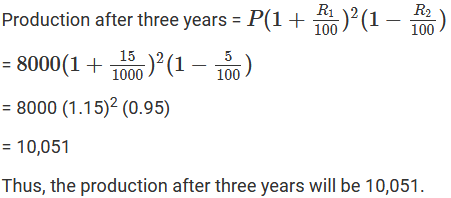Question: 15

The population of a city increases each year by 4% of what it had been at the beginning of each year. If the population in 1999 had been 6760000, find the population of the city in

(i) 2001

(ii) 1997

Solution:Question: 16

Jitendra set up a factory by investing Rs 2500000. During the first two successive years, his profits were 5% and 10% respectively. If each year the profit was on previous year’s capital, compute his total profit.

Solution:```### Course Features

• 728 Video Lectures
• Revision Notes
• Previous Year Papers
• Mind Map
• Study Planner
• NCERT Solutions
• Discussion Forum
• Test paper with Video Solution